# Choicelessness

## Problem, Set, Match

Posted in architecture, hobbies, personal, programming, projects by johnsnavely on January 11, 2009

I’m working on a little project that has to do with architecture, but I’m stuck. (Because I’m stupid at math and never took that darn linear algebra course in college.)

So I’m appealling to all my readers who, in fact, are smart. Here’s a math problem for you.

Let’s say I have a ruler and a camera. (which I do) I can take a picture like this:Fig 1 A

I’ve marked each inch with a little “x”. If we were to look just at the x’s the picture would look like this: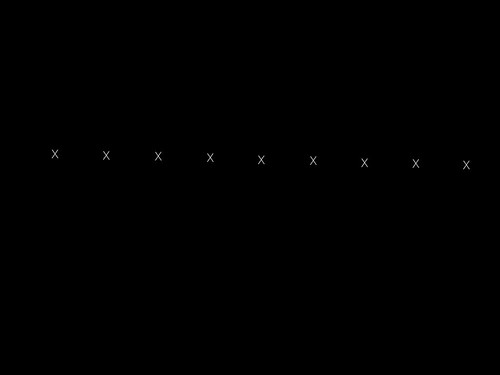Fig 1 B

Although the ponts are rotated slightly, since the ruler parallel to the base of the camera’s view frustum (ignoring lens distortion), the points are equidistant. If I wanted to figure out what kind of line this was just from these dots, I could easily fit the points to a curve (in this case a straight line) and rotate them straight. To make it look like this: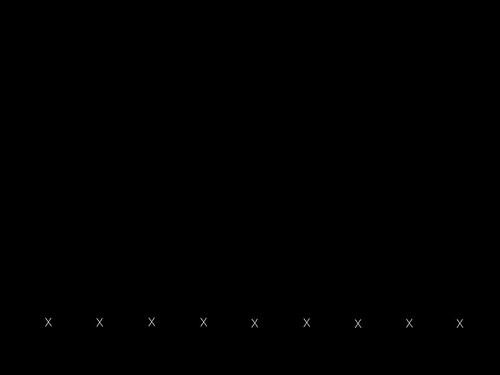Fig 2

But what about this case? Where the ruler is in perspective…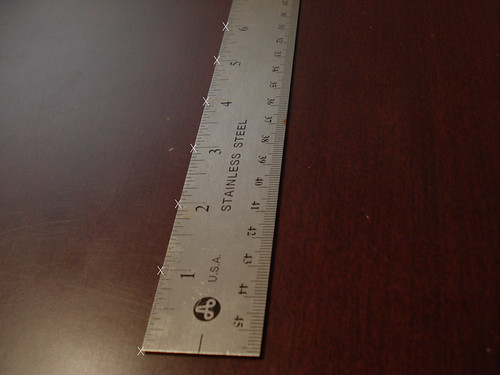Fig 3 A

The dots would look like this: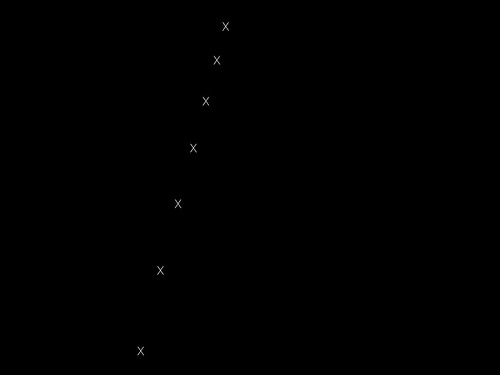Fig 3 B

Here the dots are not equidistant. However, I know that this is a straight line and since the distances shrink proportionately each time, I can again redisplay this curve on a plane (again, a straight line) like this (Fig 2):Fig 2

Now comes the hard part! What if I’ve got a ruler that looks like this?: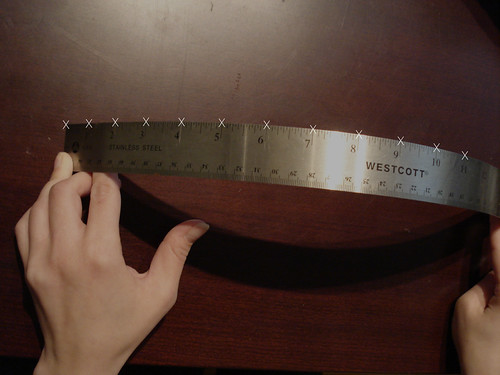Fig 4 A

Dots like this:Fig 4 B

Can I flatten those to this?:Fig 5

So the question is: Given a set of points like Fig 4B. Assuming points are equidistant, and the curve lies in a single plane (i.e. isn’t three dimensional) can you transform each of the points into a “flat” plane, where the distance between the points is actually the same?

Some issues:

1.) Since the ruler’s curved, the points are no longer exactly equidistant, but we should assume that they are. (I think, in fact, that the problem is unsolvable if we don’t.)

2.) Without more than one view or camera information, the curve could be reflected. So there are actually two solutions for every input set of points.

3.) This isn’t a 3d to 2d transformation per se, really just a 2d to 2d transformation.

Advertisements

### 3 Responses

Subscribe to comments with RSS.

1.johnsnavely said, on January 11, 2009 at 7:36 pm

Update:

I don’t think this works with a single camera. I’m going to try two and see if I can’t get it working…

2.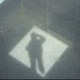Jorge Ortiz said, on January 21, 2009 at 7:46 pm

Even in your first example, the points are not really equidistant because of the (slight) curvature of the camera lens.

I don’t understand what your goal is though… Have a general transformation such that the end result is always a picture where the points are equidistant?

3.johnsnavely said, on January 21, 2009 at 11:07 pm

Yeah, let’s assume that I can correct for the lens distortion.

The general idea is that if we know some information about the object, we wouldn’t have to use stereoscopy to find position. That is, I could get 3d coordinates from a single image, instead of two. (This would be pretty useful, since most laptops only have one camera.)

Turns out I was half right. If you look at this OpenCV function, it’s possible to do if the points aren’t coplanar. Sadly, the points on the ruler are coplanar, so I’m going to have to use two cams/two images to figure out their positions.